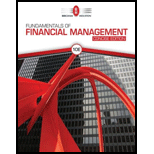# INTEREST RATE PREMIUMS A 5-year Treasury bond has a 5.2% yield. A 10-year Treasury bond yields 6.4%, and a 10-year corporate bond yields 8.4%. The market expects that inflation will average 2.5% over the next 10 years (IP 10 = 2.5%). Assume that there is no maturity risk premium (MRP = 0) and that the annual real risk-free rate, r″, will remain constant over the next 10 years. (Hint: Remember that the default risk premium and the liquidity premium are zero for Treasury securities: DRP = LP = 0.) A 5-year corporate bond has the same default risk premium and liquidity premium as the 10-year corporate bond described. What is the yield on this 5-year corporate bond?### Fundamentals Of Financial Manageme...

10th Edition
Eugene F. Brigham + 1 other
Publisher: Cengage Learning
ISBN: 9781337902571

#### Solutions

Chapter
Section### Fundamentals Of Financial Manageme...

10th Edition
Eugene F. Brigham + 1 other
Publisher: Cengage Learning
ISBN: 9781337902571
Chapter 6, Problem 17P
Textbook Problem
777 views

## INTEREST RATE PREMIUMS A 5-year Treasury bond has a 5.2% yield. A 10-year Treasury bond yields 6.4%, and a 10-year corporate bond yields 8.4%. The market expects that inflation will average 2.5% over the next 10 years (IP10 = 2.5%). Assume that there is no maturity risk premium (MRP = 0) and that the annual real risk-free rate, r″, will remain constant over the next 10 years. (Hint: Remember that the default risk premium and the liquidity premium are zero for Treasury securities: DRP = LP = 0.) A 5-year corporate bond has the same default risk premium and liquidity premium as the 10-year corporate bond described. What is the yield on this 5-year corporate bond?

Summary Introduction

To identify: The expected yield on 5-year Treasury bond.

Introduction:

Yield:

The yield is the percentage of the securities at which the return is provided by the company to its investors. Yield can be used in the form of dividend and interest.

### Explanation of Solution

In order to compute the yield on 5-year Treasury bond; the real risk-free rate of10-year Treasury bond, the inflation premium on 5-year Treasury bond, and the total value of liquidity premium and default risk premium on 10-year bond need to calculate.

Compute the real risk-free rate

Given,

The yield of 10-year Treasury bond is 6.4%.

The inflation rate at 10-year is 2.5%.

Formula to calculate the real risk-free rate derives from the formula of expected yield,

rT10=r*+IP10r*=rT10IP10

Where,

• r* is the real-risk free rate.
• rT10 is yield of treasury bonds.

Substitute 6.4% for rT10 and 2.5% for IP.

r*=6.4%2.5%=3.9% (1)

The real risk-free rate is 3.9%.

Compute the inflation premium on 5-year Treasury bond

The yield at 5-year Treasury securities is 5.2%. (Given)

The real risk-free rate is 3.9%. (Calculated in equation (1))

Formula to calculate the inflation premium derives from the formula of expectedyield,

rT5=r*+IP5IP5=rT5r*

Where,

• r* is real-risk free rate.
• rT5 is yield of treasury bonds.

Substitute 5.2% for rT5 and 3.9% for r*.

IP5=5.2%3.9%=1.3% (2)

The inflation premium of 5 year is 1.3%.

The yield of 10-year corporate bond is 8.4%. (Given)

The real risk-free rate is3.9%. (Calculated in equation (1))

The inflation premium of 10 year is 2.5%. (Given)

Formula to calculate the default risk premium, liquidity premium derives from the formula of yield,

rC10=r*+IP10+DRP10+LP10DRP10+LP10=rC10(r*+IP10)

Where,

• DRP10 is the default risk premium

### Still sussing out bartleby?

Check out a sample textbook solution.

See a sample solution

#### The Solution to Your Study Problems

Bartleby provides explanations to thousands of textbook problems written by our experts, many with advanced degrees!

Get Started

Find more solutions based on key concepts
COST OF COMMON EQUITY Percy Motors has a target capital structure of 40% debt and 60% common equity, with no pr...

Fundamentals of Financial Management, Concise Edition (with Thomson ONE - Business School Edition, 1 term (6 months) Printed Access Card) (MindTap Course List)

What is structured systems design?

Pkg Acc Infor Systems MS VISIO CD

Explain why it is easy to update a TDABC model.

Cornerstones of Cost Management (Cornerstones Series)